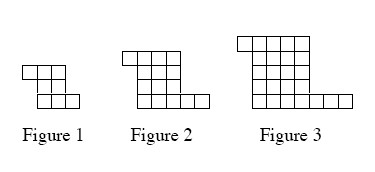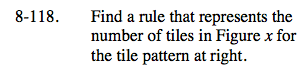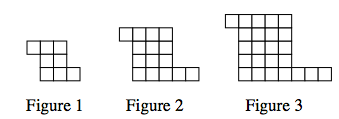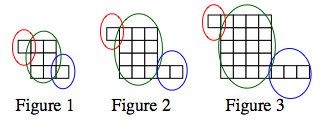### Home > CAAC > Chapter 8 > Lesson 8.3.3 > Problem8-118

8-118.

Find a rule that represents the number of tiles in Figure x for the tile pattern below. Homework Help ✎The red circles point out the left arms,
the green circles point out the rectangles,
and the blue circles point out the right arms.

Notice the following:
The length of the rectangle in Figure 1 is 3 units × 2 units;
the rectangle in Figure 2 is 4 units × 3 units;
and the rectangle in Figure 3 is 5 units × 4 units.First, look at the left arm. It remains constant in each of these figures, with a value of 1.

Let x = Figure number.
There is a rule for the size of the rectangles:
(x + 2) (x + 1),
where (x + 2) represents the length
and (x + 1) represents the width.

Now, consider the right arms. Its length matches its corresponding figure number.

y = left arm + rectangle + right arm
y = 1 + (x + 2)(x + 1) + x
Now simplify.